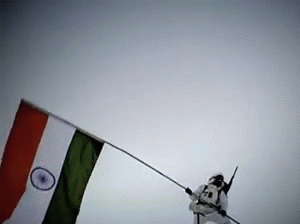### CDS, AFCAT, SSC CGL 2017 QUANT QUIZ

CDS, AFCAT, SSC CGL 2017 QUANT QUIZ

Dear candidates who are going to appear for  CDS, AFCAT, SSC CGL 2017 QUANT QUIZ exams can prepare for General Awareness Questions. Just try to read and practice such questions to clear AFCAT exams.

### CDS, AFCAT, SSC CGL 2017 QUANT QUIZ

1. The ratio of the cost price to the profit for commodity is 7 : 4. If the cost price is Rs. 35.Find the profit earned.

(a) Rs.15
(b) Rs.35
(c) Rs.30
(d) Rs.20

2. Five years ago the average age of P and Q was 20 years. The average age of P, Q and R today is 22 years. The age of R (in years) after 10 years will be

(a) 26
(b) 22
(c) 30
(d) 32

3. A man sold two TV sets for R. 2,400 each, gaining 20% on one set and losing 20% on the other set. Find his net gain or loss.

(a) gain Rs.480
(b) gain Rs.500
(c) loss Rs.200
(d) gain Rs.200

4. The average weight of three person A, B and C is 80 kg. Another man D joins the group and the average now becomes 82 kg. Another man E whose weight is 5 kg less than that of D replaces A. The average weight of B, C, D and E becomes 81 kg. The weight (in kg) of A is

(a) 88
(b) 87
(c) 86
(d) 77

5. The average of 10 numbers is 9. If each number is multiplied by 11, then the average of the new set of numbers is

(a) 90
(b) 99
(c) 20
(d) 19

6. A dishonest trader defrauds, by false balance, to the extent of 10% in buying as well as in selling his goods. His gain percent is

(a) 18%
(b) 20%
(c) 25%
(d) 21%

7. The marked price of an article is 19% higher than the cost price. A discount of 19% is given on the marked price. In this kind of sale, the seller bears

(a) a loss of 2.5%
(b) a gain of 3.59%
(c) a loss of 3.61%
(d) no loss, no gain

8. In a brass utensil, copper is 1360 gm and zinc is 240 gm. Then the percentage of copper in the utensil is
(a) 88
(b) 87
(c) 86
(d) 85

9. The cost price of an article is Rs. 81. The shopkeeper sold it gaining 10% on the selling price. The selling price of the article is

(a) Rs.86
(b) Rs.88
(c) Rs.90
(d) Rs.92

10. When 12 dozens of articles bought at Rs. 180 and are sold at Rs. 16.50 per doze, the gain percent is

(a) 8%
(b) 10%
(c) 12%
(d) 18 %

1- (d)
Profit earned =(4/7*35)
= Rs.20

2- (a)
ATQ,
(P+Q)-10 = 2*20
P + Q = 50
P + Q + R = 22*3
R = 66 - 50 = 16
Age after 10 years = 16 + 10 = 26 years

3- (c)

4- (b)

5- (b)
New average = 9*11 = 99

6- (d)

7- (c)

8- (d)

9- (c)
The selling price of article = (81*100/(100-90)) = 90

10-(b)
Cost price of article per dozen = 180/12
= 15
selling price of article per dozen = 16.50
The gain percent = (16.5-15)/15*100 = 10%

For more  CDS, AFCAT, SSC CGL 2017 QUANT QUIZ Study materials keep visiting our page for more updates or follow us on Facebook. If any more Synonyms words comment below. We would be updating our page with more  CDS, AFCAT, SSC CGL 2017 QUANT QUIZ

## .